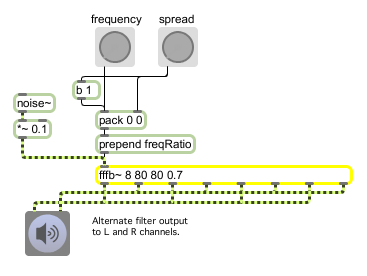fffb~

Fast fixed filter bank

Description

The fffb~ object implements a bank of bandpass filter objects, each of which is similar to the reson~ filter object. An input signal is applied to all filters, and the outputs of each filter are available separately. This object is more efficient than using a number of reson~ objects, but for the sake of speed does not accept signals for parameter changes.

Arguments

Name Type Opt Description
number-of-filters int Obligatory. The first argument specifies the number of filters.
1st-filter-frequency (float) remaining-filter-frequency-ratios (float) and general-Q-factor (float) list opt Three additional float arguments may be used to specify the frequency of the first filter, the ratio of frequencies between successive filters, and the Q factor for all of the filters.
harmonic-series-flag (H) symbol opt If you use the letter H as the second argument rather than a float, the filters will be tuned to a harmonic series rather than with ratios of frequencies.

Messages

 list filter-index and settings [list] Performs the same function as anything. anything filter-index and settings [list] A filter index followed by just a frequency or a frequency and a Q setting will set the particular filter addressed by the index number to the settings which follow it. Q bandwidths [list] In left inlet: The symbol Q, followed by a list consisting of an int and one or more floats, sets the Q factors of the filters, starting with the filter whose index is given by the first number. This filter's Q factor is set to the second number in the list. Any following numbers in the list set the Q factors of filters following the first designated one. Indices are zero-based. QAll general-bandwidth [list] In left inlet: The word QAll, followed by a float, sets the Q of all of the filters to the given floating-point value. clear Clears the fffb~ object's memory of previous inputs and outputs. freq filter-frequencies [list] In left inlet: The word freq, followed by a list consisting of an int and one or more floats, sets the center frequencies of the filters starting with the filter whose index is given by the first number. This filter's frequency is set to the second number in the list. Any following numbers in the list set the frequencies of filters following the first designated one. Indices are zero-based. For example, the message freq 3 1974.0 333.0 1234.0 sets the frequency of the fourth filter to 1974Hz, the fifth filter to 333Hz, and the sixth filter to 1234Hz. gain amplitudes [list] In left inlet: The word gain, followed by a list consisting of an int and one or more floats, sets the gains of the filters starting with the filter whose index is given by the first number. This filter's gain is set to the second number in the list. Any following numbers in the list set the gains of filters following the first designated one. Indices are zero-based. freqAll general-center-frequency [list] in left inlet: The word freqAll, followed by a float, sets the center frequencies of all of the filters to the given floating-point value. freqRatio frequency-ratios [list] In left inlet: The word freqRatio, followed by a list of two or more numbers sets the center frequency of the first filter to the first value in the list, and sets the frequencies of the remaining filters by repeatedly multiplying the first value by the second, so that the ratio of frequencies of successive filters is the second value. For example, the message freqRatio 440. 2. sets the frequency of the first filter to 440Hz, the frequency of the second to 880Hz, the frequency of the third to 1760Hz, and so on. If the second item in the list is the letter H rather than a number, the filters will be tuned in a harmonic series. For example, the message freqRatio 100 H sets the frequencies of the filters to 100Hz, 200Hz, 300Hz, 400Hz, and so on. gainAll general-amplitude [list] In left inlet: The word gainAll, followed by a float, sets the gain of all of the filters to the given floating-point value. signal The signal present at the left inlet is sent to all of the filters.

Output

signal: The output of each filter is provided at a separate outlet. The leftmost outlet is the output of the first filter.

ExamplesStereo expansion by altering the base frequency and frequency ratio